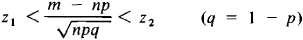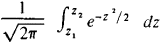Laplace Theorem

Laplace Theorem

the simplest limit theorem of the theory of probability, related to the distribution of the deviations of the frequency of occurrence of an event from its probability in independent trials. The theorem was proved in a general form by P. Laplace in his book Théorie analytique des probabilités (1812). A particular case of the Laplace theorem wasknown to A. de Moivre in 1730, so that the Laplace theorem is sometimes called the De Moivre-Laplace theorem.

The Laplace theorem is formulated as follows. Let the probability of occurrence of some event E be equal to ρ (0 < p < 1) in each of η independent trials and let m be the number of trials in which E actually occurs. Then the probability of the inequalityfor a sufficiently large number of trials differs as little as desired fromIf we denote the random variable by Xk, which takes the value 1 when E occurs in the kth trial and the value 0 when E does not occur, then m can be represented as the sum of independent random variables, that is, m = X1 + • • • + Xn. This allows us to consider the Laplace theorem as a particular case of more general limit theorems of probability theory, such as the Liapu-nov theorem.

Approximate values of the probabilities given by the Laplace theorem are used in practice as accurate values for npq on the order of tens and more.

IU. V. PROKHOROV

Site: Follow: Share:
Open / Close